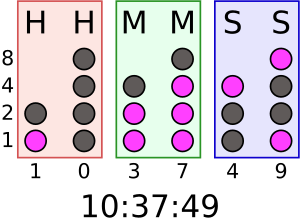# Place value facts for kids

Kids Encyclopedia Facts
(Redirected from Positional notation)A binary clock can use lamps or diodes to show the time as binary digits. The image above shows the time as a BCD code.

Place value in mathematics is the value represented by a digit

In the decimal system (which is base 10) with digits (0-9) the first column left of the decimal place is the units column, the next column to the left is the tens, the next is the hundreds. The first column right of the decimal place is the tenths column, the next is hundredths, the next is thousandths

This can be illustrated as H T U . t h th

## Images for kidsPlace value Facts for Kids. Kiddle Encyclopedia.